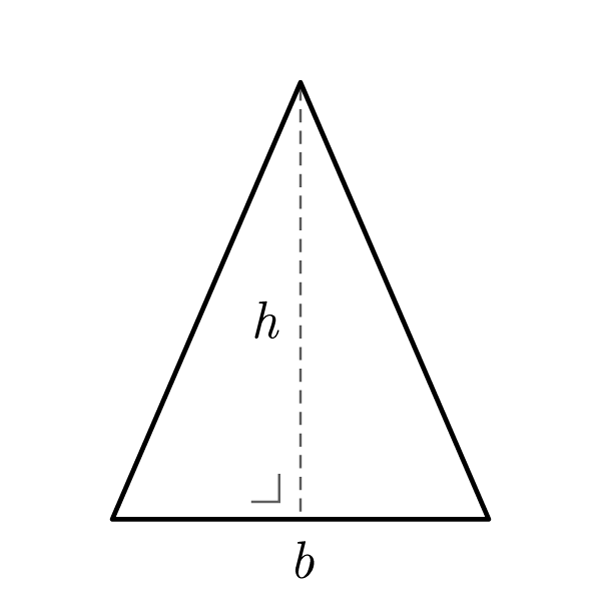# Isosceles Triangle

 Base $$b$$ Oblique Side $$S$$ Height $$h$$
$$2p = b + S \times 2$$
Perimeter
$$b = 2p - S \times 2$$
$$S = \frac{2p - b}{2}$$
$$A = \frac{b \times h}{2}$$
Area
$$b = \frac{A \times 2}{h}$$
$$h = \frac{A \times 2}{b}$$
$$S = \sqrt{ {h}^2 + {\left(\dfrac{b}{2}\right)}^2 }$$
Oblique Side (Pythagoras' theorem)
$$h = \sqrt{ {S}^2 - {\left(\dfrac{b}{2}\right)}^2 }$$
$$b = \sqrt{ {S}^2 - {h}^2 } \times 2$$

## Definition

An isosceles triangle is a triangle with two congruent sides.

## Properties

1. Two congruent sides
2. Base angles are congruent
3. All the  Generic Triangle formulas are valid
4. The height relative to the base divides the shape in two congruent right triangles. For these are valid the  Right Triangle formulas## Isosceles Triangle Formulas

Data Formula
Perimeter 2p = b + S × 2
Area A = (b × h) / 2
Oblique Side S = √[ h2 + (b / 2)2 ]
Base b = (A × 2) / h
Height h = (A × 2) / b
Base b = 2p - S × 2
Side S = (2p - b) / 2
Height h = √[ S2 - (b / 2)2 ]
Base b = √( S2 - h2 ) × 2